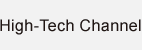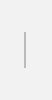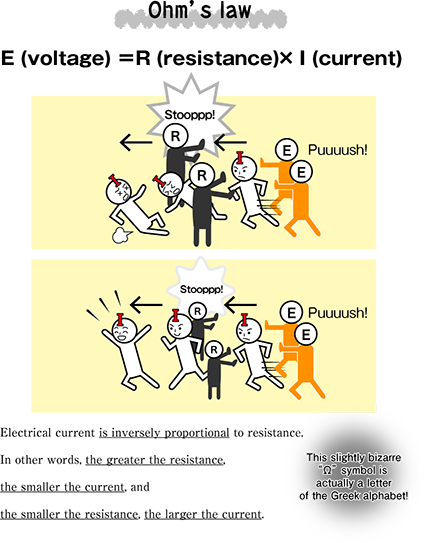### An SI-unit that disturbs electrical current

Symbol: Ω / Base data: electrical resistance. SI derived units.
Electrical resistance between two points of a conductor when a constant potential difference of 1V, applied to these points, produces in the conductor a current of 1A

Definition comes from current and voltage

“Electrical current is inversely proportional to resistance”. This is Ohm’s law. Do you remember it? “Ω”, the sign that represents electrical resistance, is an SI-derived unit. Its definition comes from electrical current and voltage. This bizarre symbol came about to differentiate “O” (first letter of Ohm) from “0” (zero).